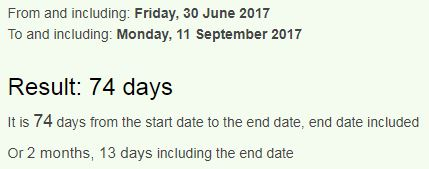## Cipher## Jun 30, 2017

### Reminder of Sarin gas attack 88 days later - 144/227

http://edition.cnn.com/2017/06/30/middleeast/syria-khan-sheikhoun-chemical-attack-sarin/index.htmlSarin used as weapon in Syria chemical attack = 10+1+9+9+5 + 3+10+5+4 + 1+10 + 5+5+1+7+6+5 + 9+5 + 10+7+9+9+1 + 3+8+5+4+9+3+1+3 + 1+2+2+1+3+2 = 193 (Single Reduction)The attack happened on "April Fourth" = 1+16+18+9+12 + 6+15+21+18+20+8 = 144 (English Ordinal)
In a "rebel-held" town of "Khan Sheikhoun" = 11+8+1+14 + 19+8+5+9+11+8+15+21+14 = 144 (English Ordinal)
rebel-held = 9+5+2+5+3 + 8+5+3+4 = 44 (Full Reduction)
Sarin gas attack = 19+1+18+9+14 + 7+1+19 + 1+20+20+1+3+11 = 144 (English Ordinal)
Chemical weapons attack = 3+8+5+30+9+3+1+20 + 900+5+1+60+50+40+90 + 1+100+100+1+3+10 = 1440 (Jewish)
Forty four = 6+15+18+20+25 + 6+15+21+18 = 144 (English Ordinal)
Assad = 1+19+19+1+4 = 44 (English Ordinal)
44 is often coded with killing and military action - Kill 44 (English Ordinal)Execution = 44 (Full Reduction)Military = 44 (Full Reduction)Genocide = 44 (Full Reduction)....

Sarin gas = 19+1+18+9+14 + 7+1+19 = 88 (English Ordinal)
eighty nine killed = 5+9+7+8+2+7 + 5+9+5+5 + 2+9+3+3+5+4 = 88 (Full Reduction)
Poison = 16+15+9+19+15+14 = 88 (English Ordinal)88 days is also 2 months and 27 days, reminding us of 227 (22/7 = 3.14..) - Twenty two divided by seven = 20+23+5+14+20+25 + 20+23+15 + 4+9+22+9+4+5+4 + 2+25 + 19+5+22+5+14 = 314 (English Ordinal)
Sarin gas = 90+1+80+9+40 + 7+1+90 = 318 (Jewish)
7/22 = 0.318... This is a very important number in the bible connecting to the second coming of Jesus Christ, the Sun of God, also the Sun in Greek carries this number
Ηλιος = 8+30+10+70+200 =318GB -> G is the 7th letter, B is the 2nd so we can read this as 72 just like "Syria" = 19+25+18+9+1 = 72 (English Ordinal)

Sarin = 8+26+9+18+13 = 74 (Reverse Ordinal)
poison = 11+12+18+8+12+13 = 74 (Reverse Ordinal)
They are reminding us of this attack 74 days before al-Assad's birthday which is September Eleventh - Assad = 1+90+90+1+4 = 186 (Jewish)If we don't count the end date we see 73 days to his birthday measuring from a date with 73 numerology - 6/30/2017 = 6+30+20+17 = 73
73 is the 21st prime number and Assad's birthday this year lands on a date with 21 numerology (9+1+1+2+0+1+7 = 21)